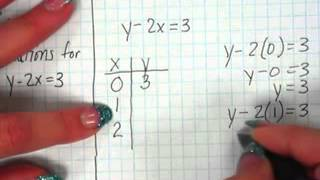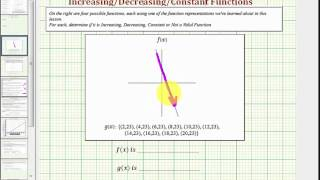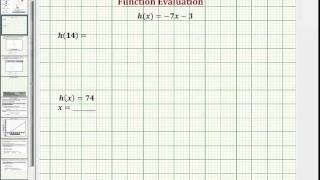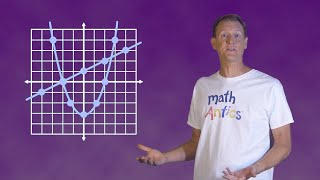# 7.1 Graphing Ordered Pairs

##By TeacherTube Math

YouTube presents 7.1 Graphing Ordered Pairs an educational video resource on math.# Ex: Determine if a Function is Incr, Decr, or Constant from Graph, Table, and Ordered Pairs

##By Mathispower4u

Ex: Determine if a Function is Incr, Decr, or Constant from Graph, Table, and Ordered Pairs# Ex: Find Function Output and Input Using a Graph, Table, Ordered Pairs, and Function Rule

##By Mathispower4u

Ex: Find Function Output and Input Using a Graph, Table, Ordered Pairs, and Function Rule# Graphing points

##By Khan Academy

This exercise asks us to graph a set of points from an ordered pair on the x and y axis of a coordinate plane.# Graphing points

##By Khan Academy

This exercise asks us to graph a set of points from an ordered pair on the x and y axis of a coordinate plane.# Points on the coordinate plane

##By Khan Academy

Some of the given ordered pairs are already graphed on the coordinate pane in this example, but not all of them. Can you tell which haven't?# Points on the coordinate plane

##By Khan Academy

Some of the given ordered pairs are already graphed on the coordinate pane in this example, but not all of them. Can you tell which haven't?# Points on the coordinate plane

##By Khan Academy

Some of the given ordered pairs are already graphed on the coordinate pane in this example, but not all of them. Can you tell which haven't?# Points on the coordinate plane

##By Khan Academy

Some of the given ordered pairs are already graphed on the coordinate pane in this example, but not all of them. Can you tell which haven't?# Constraint solution sets of two-variable linear inequalities

##By Khan Academy

Sal determines which x-values make the ordered pair (x,-7) a solution of 2x-7y<25. He also solves a similar problem where the inequality is given as a graph.# Visualizing and interpreting relationships between patterns

##By Khan Academy

We're building in complexity as we interpret and graph the relationships between patterns in the given ordered pairs. Don't stress. We're here to help!# Khan Academy: Linear Equation Application Problem

##By Khan Academy

Get more practice with functions by watching this word problem. Here you must graph the equation and use it to find a specific output. Start by creating a table to get a range of values. You'll need several ordered pairs to create the graph!# Constraint solution sets of systems of linear inequalities

##By Khan Academy

Given the graph of a system of inequalities, Sal finds the x-values that make the ordered pair (x,-2) a solution of the system, which is the solution set constrained to y=-2. Then he solves a similar problem where x is constrained to 4.# Algebra Basics: Graphing On The Coordinate Plane - Math Antics

##By mathantics##By Lumos Learning

8th grade math lesson addressing Common Core Standards (Massachusetts Curriculum Framework Standard 8.EE.6).##By

8th grade math lesson addressing Common Core Standards (Massachusetts Curriculum Framework Standard 8.EE.6).# Algebra Basics: What Are Functions? - Math Antics

##By mathantics# High School Math

##By

High school math lesson.# Autism: An evolutionary perspective, Professor Simon Baron-Cohen, 1st Symposium of EPSIG, 2016

##By Lumos Learning

First Symposium of the Evolutionary Psychiatry Special Interest Group of the Royal College of Psychiatrists, Oct 4th 2016 in London. Lecture by Professor Simon Baron-Cohen from Cambridge University Autism Research Centre.